Pearson Product-Moment Correlation

Subsections:

The Pearson product-moment correlation is a parametric measure of association for two variables. It measures both the strength and the direction of a linear relationship. If one variable X is an exact linear function of another variable Y, a positive relationship exists if the correlation is 1 and a negative relationship exists if the correlation is1. If there is no linear predictability between the two variables, the correlation is 0. If the two variables are normal with a correlation 0, the two variables are independent. However, correlation does not imply causality because, in some cases, an underlying causal relationship might not exist.

The scatter plot matrix in Figure 2.4 displays the relationship between two numeric random variables in various situations.

Figure 2.4: Correlations between Two Variables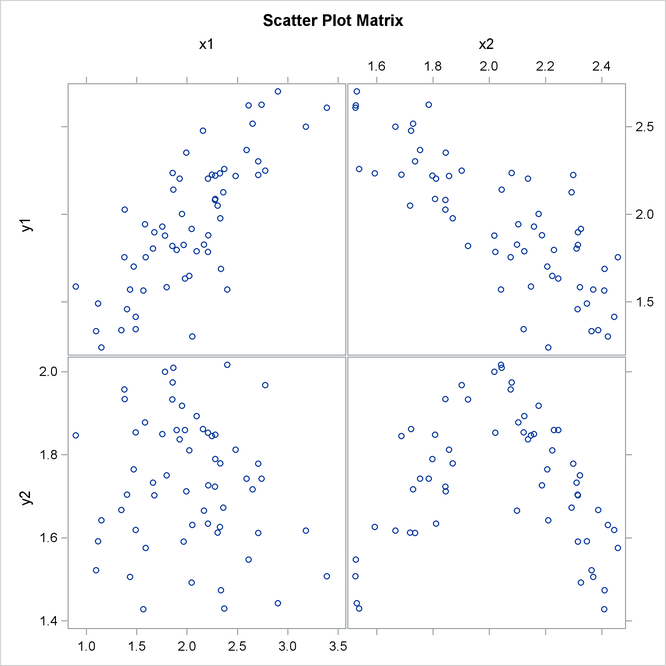The scatter plot matrix shows a positive correlation between variables Y1 and X1, a negative correlation between Y1 and X2, and no clear correlation between Y2 and X1. The plot also shows no clear linear correlation between Y2 and X2, even though Y2 is dependent on X2.

The formula for the population Pearson product-moment correlation, denoted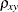, is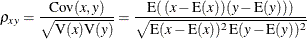The sample correlation, such as a Pearson product-moment correlation or weighted product-moment correlation, estimates the population correlation. The formula for the sample Pearson product-moment correlation is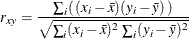where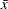is the sample mean ofand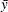is the sample mean of
. The formula for a weighted Pearson product-moment correlation is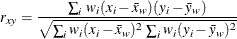where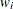is the weight,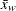is the weighted mean of, and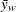is the weighted mean of.

Probability Values

Probability values for the Pearson correlation are computed by treating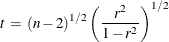as coming from a t distribution with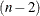degrees of freedom, whereis the sample correlation.